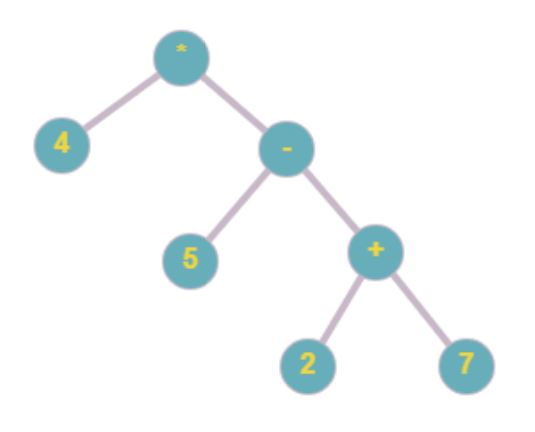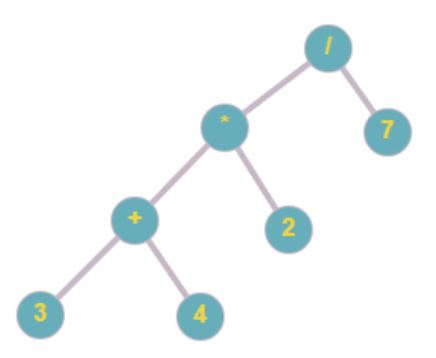2

# Ninja's Encryption ll

Difficulty: MEDIUMContributed By
Omkar Deshmukh
Avg. time to solve
15 min
Success Rate
85%

Problem Statement

#### So your task is to write the code that can evaluate the given expression in the form of a binary tree where each node must have two children except leaf nodes and leaf nodes represent integer while other nodes represent binary operators like ‘-’, ‘+’, ‘/’, ‘*’.

##### Example:``````Consider the following binary search tree so the evaluation of the expression is ( 4 *  ( 5 - ( 2+ 7 ) ) ) = ‘-16’
``````

#### Note:

``````You have been given the root of the tree.
``````
##### Input Format:
``````The first line contains an integer  ‘T' which denotes the number of test cases or queries to be run. Then the test cases follow.

The first line of each test case contains the elements of the tree in the level order form separated by a single space.

If any node does not have a left or right child, take -1 in its place. Refer to the example below.

Example:

Elements are in the level order form. The input consists of values of nodes separated by a single space in a single line. In case a node is null, we take -1 in its place.

For example, the input for the tree depicted in the below image would be :
````````````1
2 3
4 -1 5 6
-1 7 -1 -1 -1 -1
-1 -1

Explanation :
Level 1 :
The root node of the tree is 1

Level 2 :
Left child of 1 = 2
Right child of 1 = 3

Level 3 :
Left child of 2 = 4
Right child of 2 = null (-1)
Left child of 3 = 5
Right child of 3 = 6

Level 4 :
Left child of 4 = null (-1)
Right child of 4 = 7
Left child of 5 = null (-1)
Right child of 5 = null (-1)
Left child of 6 = null (-1)
Right child of 6 = null (-1)

Level 5 :
Left child of 7 = null (-1)
Right child of 7 = null (-1)

The first not-null node (of the previous level) is treated as the parent of the first two nodes of the current level. The second not-null node (of the previous level) is treated as the parent node for the next two nodes of the current level and so on.

The input ends when all nodes at the last level are null (-1).
``````
##### Note :
``````The above format was just to provide clarity on how the input is formed for a given tree.

The sequence will be put together in a single line separated by a single space. Hence, for the above-depicted tree, the input will be given as:

1 2 3 -1 5 6 7 -1 -1 -1 -1
``````
##### Output Format :
``````For each test case, print the evaluation of each expression

Print output of each test case in a separate line.
``````
##### Note:
``````You are not required to print anything explicitly. It has already been taken care of. Just implement the function.
``````
##### Constraints:
``````1 <= T <= 10^2
1 <= N <= 3*10^3
0 <= data <= 10^3 or ( ‘+’, ‘-’, ‘*’, ‘/’ )

Where ‘T’ is the total number of test cases, ‘N’ is the number of nodes of the tree, and data is the value mentioned on the node.

Time Limit: 1 sec
``````
##### Sample Input 1:
``````2
* 4 - -1 -1 5 + -1 -1 2 7 -1 -1 -1 -1
/ * 7 + 2 -1 -1 3 4 -1 -1 -1 -1 -1 -1
``````
##### Sample Output 1:
``````-16
2
``````

#### Explanation For Sample Input 1:

``````Test case 1:

The binary tree according to the test case is:
````````````consider the following binary search tree so the evaluation of the expression is ( 4 *  ( 5 - ( 2+ 7 ) ) ) = ‘16’

Test case 2:

The binary tree according to the test case is:
````````````consider the following binary search tree so the evaluation of the expression is ( 3 + 4 ) * 2 ) / 7 ) = ‘2’.
``````

#### Sample Input 2:

``````2
+ * - 5 4 100 20 -1 -1 -1 -1 -1 -1 -1 -1
+ * - 5 4 100 / -1 -1 -1 -1 -1 -1 20 2 -1 -1
``````

#### Sample Output 2:

``````100
110
``````Console